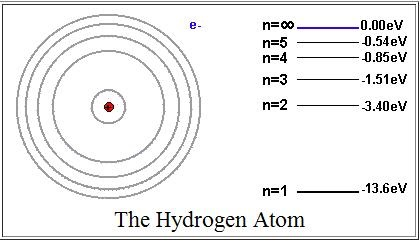# Energetic ElectronsIn atoms, electrons jump between energy levels, and they absorb or radiate energy in the form of light (photons). An electron falls to a lower energy level when it radiates a photon, and jumps to a higher energy level when it absorbs a photon.

Find the wavelength of the photon that radiates when an electron jumps from the $n = 3$ energy level down to the $n = 2$ energy level. Where is this photon in the electromagnetic spectrum?

Assumptions

• $h$ (Planck's constant) = $4.14 \times 10^-15$ eV s.
• $c$ (Speed of light) = $3 \times 10^8$ m/s.
• All values are approximate.
• Assume that this is a hydrogen atom.
×

Problem Loading...

Note Loading...

Set Loading...